## Pages

### MS 2. Weighted Average

Lets say that there are two student making several measurement on a system in two different laboratories. We can then ask how can we then relate those two measurement to a single number to represent an average measurement. At first insight it might seem trivial, Why not just add and divide it by half, the normal averaging method for two given measurement. Well, the problem with that method is that it is correct only if we assume that we have given equal weight to the measurement that we made. The question then boils down to how do we find an average given some weight on each of these measurements.

The crucial point in solving this problem would be to assume that both of these measurement are governed by the gaussian distribution. Just to be clear, the type of measurement that each of the student makes would be something like ($x_{a}\pm \sigma_{a}$) and  ($x_{b}\pm \sigma_{b}$) where $\sigma's$ are the standard deviation. It is important to keep in mind that if the discrepancy between the two measurements is much larger than both uncertainties, then it is wise to acknowledge that somethings has gone wrong in one of the measurements. It is then better to check that there were no systematic errors during the measurement.

If now we consider the true value of $x$ by $X$. Then, the probability of student obtaining a particular value $x$ is,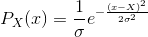where, the subscript $X$ indicates explicitly that these probabiliteis depends on the some unknown true value. Now if students find that their measurement are ($x_{a}\pm \sigma_{a}$) and  ($x_{b}\pm \sigma_{b}$) with respect to some actual value. Then the proability that each student measures each ($x_{a}\pm \sigma_{a}$) and  ($x_{b}\pm \sigma_{b}$) is just the product of the two probabilities. i.e.

Then the best estimate for the unknown true value $X$ is that value for which $x_{a},_{b}$ are most likely. This implies that the probability should then be maximum. So to find the maximum value to $P_{X}(x)$  we differentiate the above equation with respect to $X$ and setting it to zero. We then get as follows,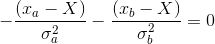which can be rewritten as,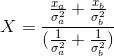This is then the best estimate of the given any two measurement. Also it can be further written as,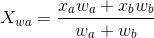where, $w_{a}=\frac{1}{\sigma_{a}^{2}}$ and $w_{b}=\frac{1}{\sigma_{b}^{2}}$ are the defined weight for the measurement given. The uncertainty is then given by as follows,

which reduces to,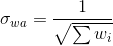This is the uncertainty of the weighted average method.

**EXAMPLE:
Say two students measure the the following measurement:
($12\pm 2$) and  ($11\pm3$), find the weighted average and the uncertainty in the weighted average.

**Anwer: $X_{wa}$=$11.69 \pm 6/\sqrt{13}$